# Math in Focus Grade 5 Chapter 8 Practice 3 Answer Key Rewriting Decimals as Fractions and Mixed Numbers

Practice the problems of Math in Focus Grade 5 Workbook Answer Key Chapter 8 Practice 3 Rewriting Decimals as Fractions and Mixed Numbers to score better marks in the exam.

## Math in Focus Grade 5 Chapter 8 Practice 3 Answer Key Rewriting Decimals as Fractions and Mixed Numbers

Rewrite each decimal as a fraction or mixed number in simplest form.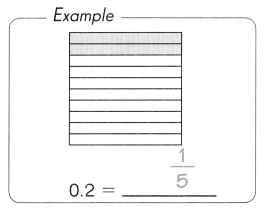Question 1.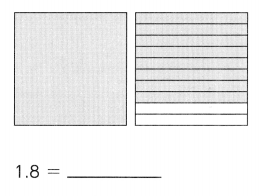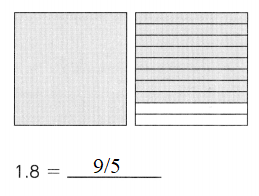1.8 in fraction is
1.8 = 9/5

Question 2.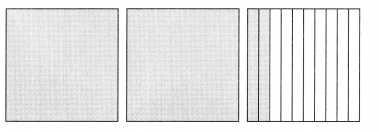2.2 = ______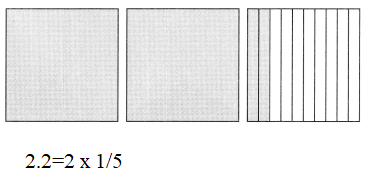2.2 in mixed number is 2 × 1/5

Question 3.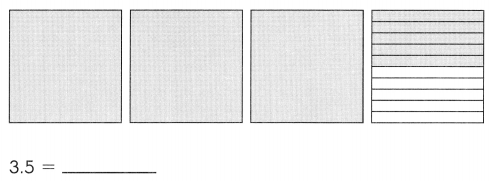3.5 mixed number is 3×1/2

Question 4.
0.36
0.36 in fraction is 9/25

Question 5.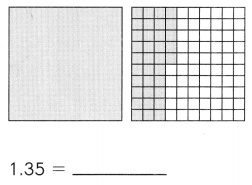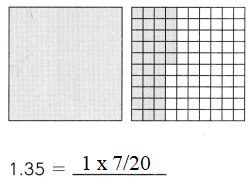1.35 in mixed number is 1×7/20

Rewrite each decimal as a fraction or mixed number in simplest form.

Question 6.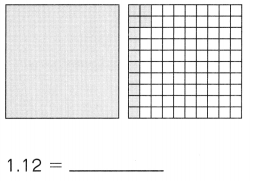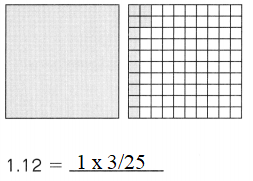1.12 in mixed number is 1×3/25

Question 7.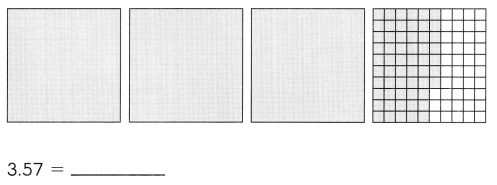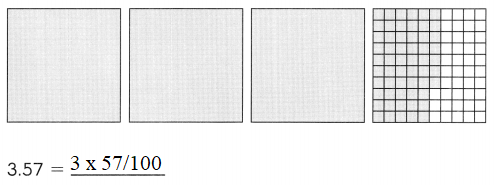3.57 in mixed number is 3×57/100

Question 8.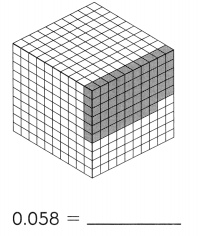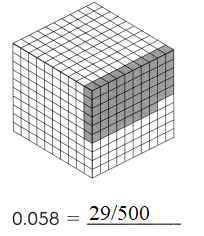0.058 in fraction number is 29/500.

Question 9.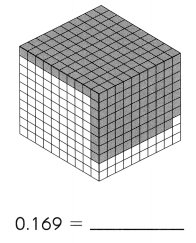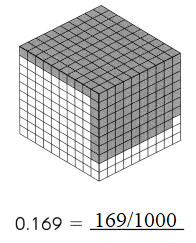0.169 in fraction number is 169/1000.

Question 10.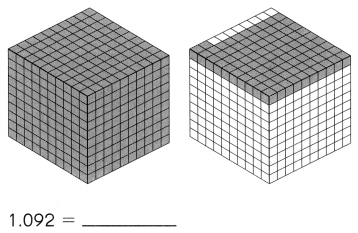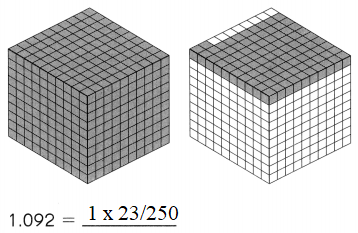1.092 in mixed number is 1×23/250.

Rewrite the decimal as a mixed number in simplest form.

Question 11.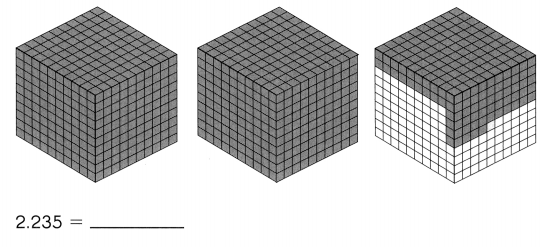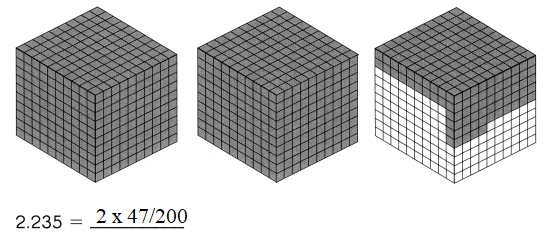2.235 in mixed number is 2×47/200.

Rewrite each decimal as a fraction or mixed number in simplest form.

Question 12.
7.3
7.3 in fraction number is 73/10.

Question 13.
26.9
26.9 in mixed number is 269/10.

Question 14.
0.59
0.59 in fraction number is 59/100.

Question 15.
15.82
15.82 in mixed number is 15×41/50.

Question 16.
1.28
1.28 in mixed number is 1×7/25.

Question 17.
4.109